# Learn About Powerful Functions In A Python GUI Windows App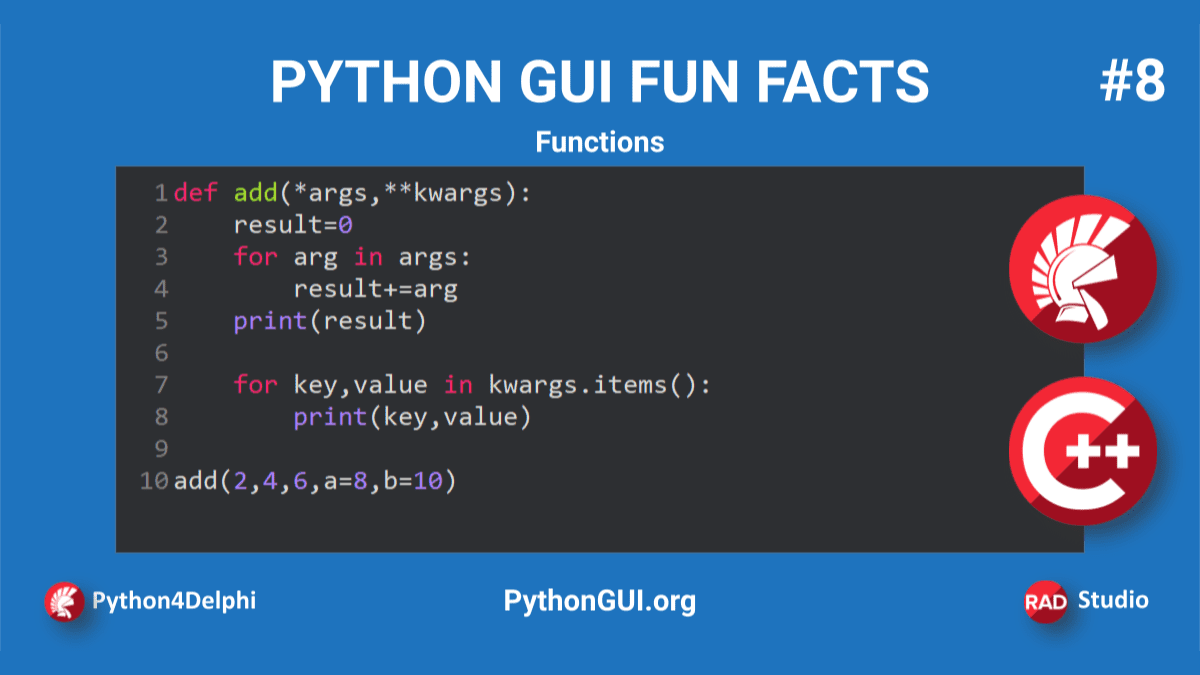Python is object-oriented but also supports functional programming. This post will demonstrate how to run another Python feature in Python4Delphi with RAD Studio:  Functions in Python GUI and gets the output. We will learn about Python functions and how to create them and call them.

A function is a block of code that has a name and we can call it. Instead of writing something 100 times, we can create a function and then call it 100 times. We can call it anywhere and anytime in our program. Functions adds reusability and modularity to our code.

Functions can take arguments and return values. You can pass data, known as parameters, into a function. A function can return data as a result.

Prerequisites: Before we begin to work, download and install the latest Python for your platform. Follow the Python4Delphi installation instructions mentioned here. Alternatively, you can check out the easy instructions found in this video Getting started with Python4Delphi.

First, open and run our Python GUI using project Demo1 from Python4Delphi with RAD Studio. Then insert the script into the lower Memo, click the Execute button, and get the result in the upper Memo. You can find the Demo1 source on GitHub.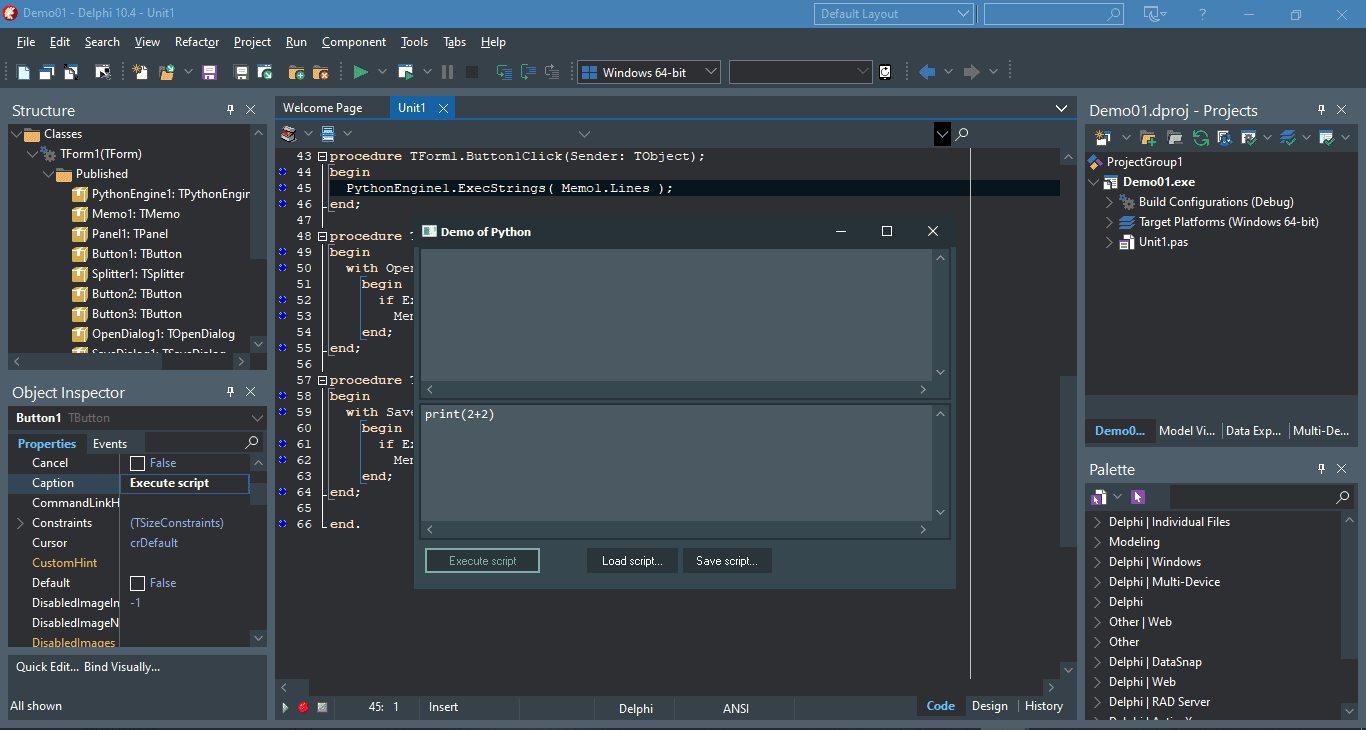Open Demo01.dproj

## 1. Creating a Function in Python

To define a function, you use the def keyword. You give the function a name and it can take some parameters if you want. Then you specify a block of code that will execute when you call the function. There are no curly braces in Python and so you need to indent this code block otherwise it won’t work. You can make the function return a value.

Define the following function to our lower Memo in Python GUI:

No expected output in our Python GUI: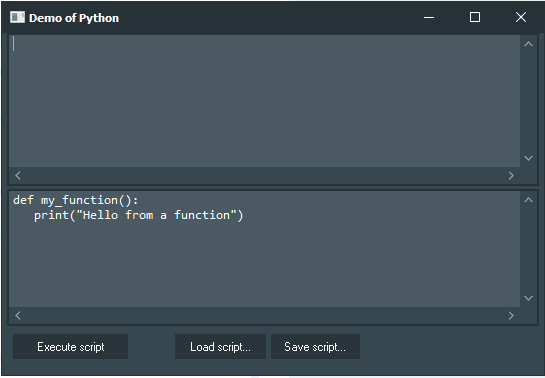Create Function in Python using Python GUI from Python4Delphi.

To expect the output, you need to call the function first.

## 2. Calling a Function in Python

To call a function, use the function name followed by parenthesis:

Output in the Python GUI upper Memo:Calling Function in Python using Python GUI from Python4Delphi.

## 3. Python Function Parameters

Functions can take values and operate on them. Let’s create another function:

In the above example, the add() function takes parameters a and b.

Python has 3 types of parameters or arguments other than positional/required arguments – default, keyword, arbitrary.

### 3.1. Default Arguments

We can specify a default value for arguments. If the user calls the function, they can skip providing a value for that argument. The default value is used.

Here b is 4 by default. So it returns 2+4, which is 6. Functions can have any number of default arguments.

Let’s see the output: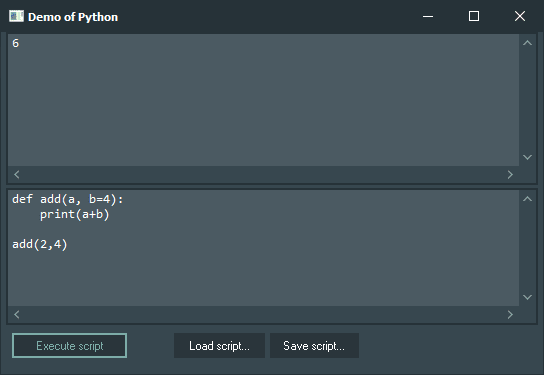Learn Default Argument in Python using Python GUI from Python4Delphi.

### 3.2. Keyword Arguments

If you pass keyword arguments to a function, you don’t need to remember the order of the parameters. Insert the following function to our Python GUI (lower Memo):

Let’s see the output: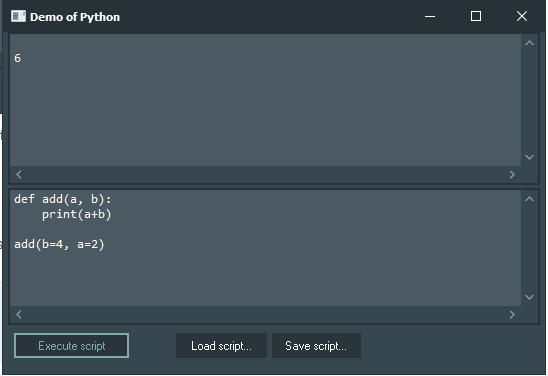Learn Keyword Argument in Python using Python GUI from Python4Delphi.

### 3.3. Arbitrary Arguments

If you don’t know how many arguments your function will get at runtime, you can use the arbitrary arguments *args and **kwargs.

*args is a variable number of arguments and **kwargs is a variable number of keyword arguments. You can call them anything.

Try the following function to our Python GUI (lower Memo):

Output: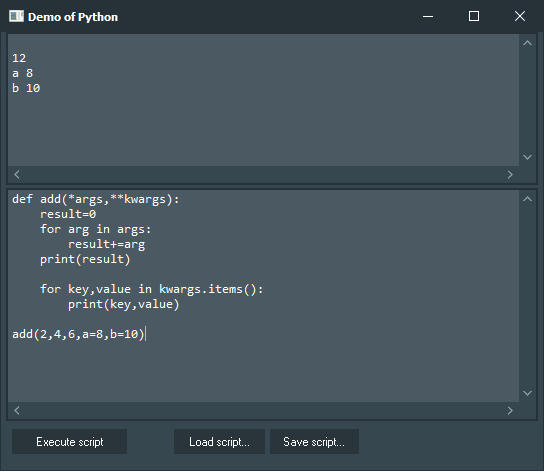Learn Arbitrary Arguments in Python using Python GUI from Python4Delphi.

2, 4, 6, are in *args and a=8 and b=10 are in **kwargs. result is 12.

## 4. Recursion in Python

When a function calls itself, it is recursion. In other words, when a function body has calls to the function itself, it is recursion. For recursion, you should specify a base condition otherwise the function can execute infinitely. Let’s take the example of calculating factorial of 5:

Output:Learn Recursion in Python using Python GUI from Python4Delphi.

Congratulations! You’ve created your own calculator to calculate factorials, using Python4Delphi.

We have learned about functions, creating them, calling them, parameters, and recursion.

Check out Python4Delphi which easily allows you to build Python GUIs for Windows using Delphi.